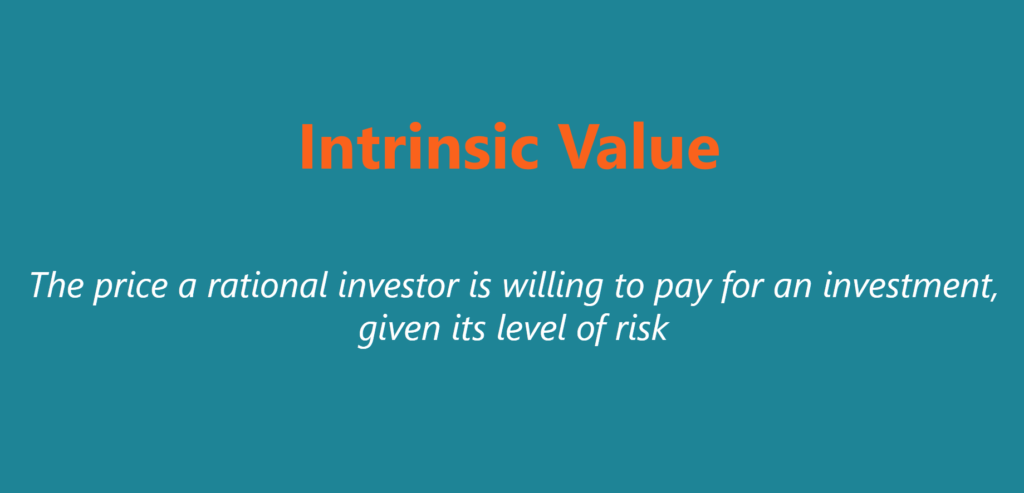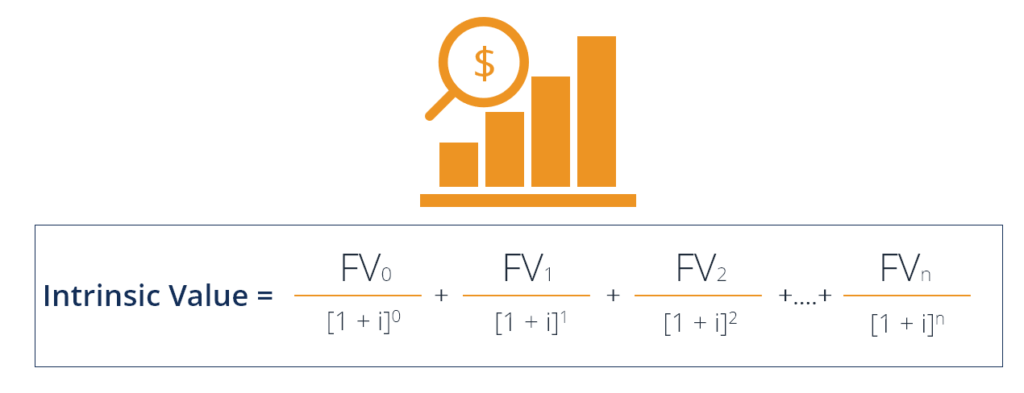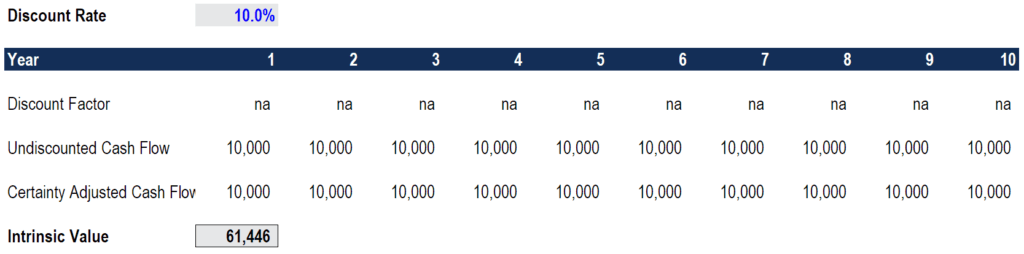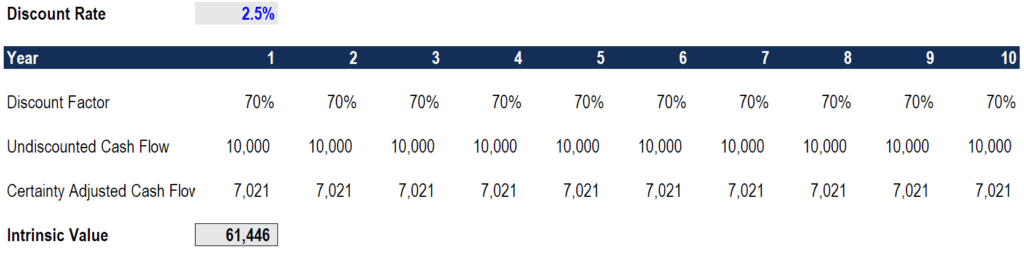# Intrinsic Value

The price a rational investor is willing to pay for an investment, given its level of risk

## What is Intrinsic Value?

The intrinsic value of a business (or any investment security) is the present value of all expected future cash flows, discounted at the appropriate discount rate. Unlike relative forms of valuation that look at comparable companies, intrinsic valuation looks only at the inherent value of a business on its own.Another way to define intrinsic value is simply, “The price a rational investor is willing to pay for an investment, given its level of risk.”

### Background

Benjamin Graham and Warrant Buffet are widely considered the forefathers of value investing, which is based on the intrinsic valuation method. Graham’s book, The Intelligent Investor, laid the groundwork for Warren Buffett and the entire school of thought on the topic.

The term intrinsic means the essential nature of a something. Synonyms include innate, inherent, native, natural, deep-rooted, etc.

### Intrinsic Value Formula

There are different variations of the intrinsic value formula but the most “standard” approach is similar to the net present value formula.Where:

NPV = Net Present Value

FVj = Net cash flow for the j th period (for the initial “Present” cash flow, j = 0

i = annual interest rate

n = number of periods included

Variations include multi-stage growth models and assigning a probability or level of certainty to the cash flows and playing around with the discount rate.

### Risk Adjusting the Intrinsic Value

The task of risk adjusting the cash flows is very subjective and a combination of both art and science.

There are two main methods:

1. Discount rate – Using a discount rate that includes a risk premium in it to adequately discount the cash flows
2. Certainty factor – Using a factor on a scale of 0-100% certainty of the cash flows in the forecast materializing (This approach is believed to be used by Warren Buffett. Learn more by reading Buffett’s annual letters to shareholders)

### Discount Rate

In the discount rate approach, a financial analyst will typically use a company’s weighted average cost of capital (WACC). The formula for WACC includes the risk-free rate (usually a government bond yield) plus a premium based on the volatility of the stock multiplied by an equity risk premium. Learn all about the WACC formula here.

The rationale behind this approach is that if a stock is more volatile, it’s a riskier investment. Therefore, a higher discount rate is used, which has the effect of reducing the value of cash flow that would be received further in the future (because of the greater uncertainty).

### Certainty Factor

A certainty factor, or probability, can be assigned to each individual cash flow or multiplied against the entire net present value (NPV) of the business as a means of discounting the investment. In this approach, only the risk-free rate is used as the discount rate, since the cash flows are already risk adjusted.

For example, the cash flow from a US Treasury note comes with a 100% certainty attached to it, so the discount rate is equal to yield, say 2.5% in this example. Compare that to the cash flow from a very high-growth and high-risk technology company. A 50% probability factor is assigned to the cash flow from the tech company and the same 2.5% discount rate is used.

At the end of the day, both methods are attempting to do the same thing – to discount an investment based on the level of risk inherent in it.

### Calculating Intrinsic Value in Excel

Below we will provide examples of how to calculate the intrinsic value in Excel using the two methods described above.

#### 1. Discount Rate

In the screenshot below, you can see how this approach is taken in Excel. The risk-adjusted discount rate for this investment is determined to be 10.0% based on its historic price volatility. In this method, there is no certainty or probability factor assigned to each cash flow, since the discount rate does all the risk adjusting.As you will see, for an investment that pays \$10,000 at the end of each year for 10 years with a 10% discount rate, the intrinsic value is \$61,446.

#### 2. Certainty Factor

In the second screenshot below, you can see how this alternative approach is taken in Excel. This time, an analyst uses only the risk-free rate of 2.5% as the discount rate. There is, however, an additional adjustment factor of 70% applied to each cash flow. The way to think about this is “there is a 70% chance of receiving \$10,000 each year”, or, “there is a 100% chance of receiving \$7,021 each year.”As you can see, for this same investment that pays \$10,000 at the end of each year for 10 years with a 70% confidence factor and 2.5% discount rate, the intrinsic value is \$61,446 (the same as method #1).

### Challenges with Intrinsic Value

The trouble with calculating intrinsic value is it’s a very subjective exercise. There are so many assumptions that must be made, and the final net present value is very sensitive to changes in those assumptions.

Each of the assumptions in the WACC (beta, market risk premium) can be calculated in different ways, while the assumption around a confidence/probability factor is entirely subjective.

Essentially, when it comes to predicting the future, it is by definition, uncertain. For this reason, all of the most successful investors in the world can look at the same information about a company and arrive at totally different figures for its intrinsic value.

### Other Forms of Valuation

Intrinsic valuation is often used for long-term investment strategies, but there are many other approaches to valuation and investing. Alternatives include technical analysis, relative valuation, and cost approach.

#### 1. Technical Analysis

Technical analysis involves looking at charts and evaluating various indicators that may signal a stock is going to go up or down in the short to medium term. Examples including candlestick charts, momentum and moving averages, relative strength, and more.

#### 2. Relative Valuation

Relative valuation looks at what other investors are willing to pay for a similar investment and assumes that they would pay a comparable price for the company in question.  The two most common examples of this are comparable company analysis (“Comps”) and precedent transactions analysis (“Precedents”).

#### 3. Cost Approach

In the cost approach, an investor looks at what the cost to build or create something would be and assumes that is what it’s worth. They may look at what it cost other to build a similar business, and take into account how costs have changed since then (inflation, deflation, input costs, etc.).

### Video Explanation of Intrinsic Value

Watch this short video to quickly understand the main concepts covered in this guide, including what intrinsic value is, the formula, how to risk adjust intrinsic value, and how to perform the calculation in Excel.

Thank you for reading this guide to intrinsic value. Hopefully, by now, you’ve gained a better understanding of how investors determine what an investment is worth to them.

CFI is the global provider of the Financial Analyst Certification in financial modeling and valuation. To continue learning and advancing your career, these additional resources will be helpful:

• The Analyst Trifecta
• Valuation Infographic
• Financial Modeling Guide
• All Valuation Articles

### Valuation Techniques

Learn the most important valuation techniques in CFI’s Business Valuation course!

Step by step instruction on how the professionals on Wall Street value a company.

Learn valuation the easy way with templates and step by step instruction!